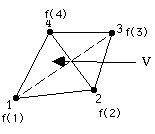III. Refine or Derefine Tets:

Each tetrahedron is tested separately for refinement or derefinement, where V is the volume of the tetrahedron.Criteria:

1) Junction: Refine if any of the tets field values straddle c. example: For c = 0 refine if f changes sign between any of the four nodes.

2) Constant: Refine if any of the tets field values exceed c. Tag for refinement if f(1) c or f(2) c or f(3) c or f(4) c

3) Maxsize: Refine if the tet volume exceeds c. Tag if V c

4) Aspect Ratio: Refine if the tets aspect ratio is less than c. For the tet the aspect ratio (AR) is defined as the ratio of the radius of the inscribed sphere of the tet to the radius of the circumscribed sphere. We renormalize this ratio by multiplying by three so that the ratio equals one for a regular tetrahedron (composed of equilateral triangular faces).

AR = 3 RIN/ROUT, where

RIN radius of inscribed sphere ROUT radius of circumscribed sphere AR is never greater than one. Tag if AR < c. Generally the smaller AR is, the more elongated the tet is.

5) Lambda Refine: Refine if lamda/dx c . Where lambda is taken to be the radius of the circumscribed sphere ROUT of the tet.

lambda = |f(xcen)| /grad f f(xcen) = (f(1) + f(2) + f(3) + f(4))/4 where xcen is the centroid of the tet, and we have assumed linear interpolations of f. grad f is evaluated for the tet by an approximation involving linear interpolation au the surface integral over the surface of the tet.